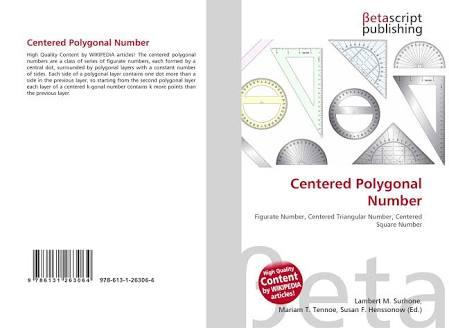# What Do You Know About The Centered Polygonal Numbers?

10 Questions | Total Attempts: 112SettingsThe centered polygonal numbers can be referred to as a class or series of figurate numbers. Each of these numbers is formed by a central dot, which is usually surrounded by a polygonal layer and has a constant number of sides. Number arrangement using this method can be made into a series of shapes and they include triangle, squares, pentagons and so on. This quiz will help you learn more about the centered polygon numbers.

• 1.
Where did arranging numbers into regular shapes like squares originate from?
• A.

Ancient Rome

• B.

Italy

• C.

Greece

• D.

Egypt

• 2.
What is the dimension of the centered polygonal numbers?
• A.

Two dimensions

• B.

Three dimensions

• C.

Four dimensions

• D.

Five dimensions

• 3.
In the centered polygonal numbers, the sequence follows a pattern; which is the pattern?
• A.

Multiple of the triangular number plus 3

• B.

Multiple of the triangular number plus 4

• C.

Multiple of the triangular number plus 1

• D.

Multiple of the tetragonal number plus 1

• 4.
In triangular numbers, which of these sequences is correct?
• A.

1, 4, 10, 19...

• B.

3, 6, 9, 12...

• C.

1, 3, 6, 12...

• D.

1, 3, 6, 10...

• 5.
How are the polygons in the centered polygon numbers are usually drawn?
• A.

Centered about a point within the polygon

• B.

At the polygon vertex

• C.

Outside the polygon

• D.

Anywhere inside the polygon

• 6.
How do we write the formula for representing the centered K-gonal number?
• A.

C_(k,n=kn/2(n-1)+1)

• B.

C_(k,n=kn/2(n+1)+1)

• C.

C_(k,n=kn/2(n-1)+1k)

• D.

C_(k,n=kn/2 (n-1)-1)

• 7.
A particular category of numbers is predominant in the centered polygonal numbers. Which of the following is this category?
• A.

Odd numbers

• B.

Prime numbers

• C.

Even numbers

• D.

Whole numbers

• 8.
In square numbers, what is the sequence?
• A.

4, 8, 16, 32...

• B.

4, 16, 64, 256...

• C.

1, 5, 13, 25...

• D.

1, 5, 9, 13...

• 9.
What is the formula to calculate a centered decagonal?
• A.

3n (n+1) - 4

• B.

5n (n+1) - 1

• C.

5n (n+1) + 1

• D.

10n (n-1) + 1

• 10.
Who proved the polynomial number theorem?
• A.

Joseph LaGrange

• B.

Fermat

• C.

Bermoulli

• D.

Berno Cauchy

Related TopicsBack to top